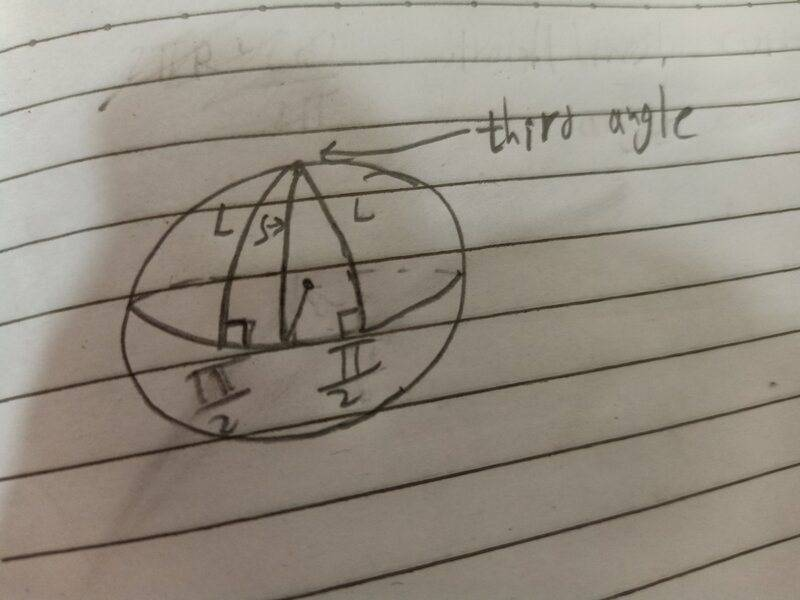# Triangles on spheres

• I
• Ahmed1029
In summary, the author argues that if a triangle has two angles of 90 degrees each, it is isosceles. If the triangle has more than two angles of 90 degrees, it is not a polygon and has only one side that is a length of a quarter of a great circle on the sphere.f

#### Ahmed1029

If I have a triangle on a sphere with two of its angles 90 degrees each, do I conclude that it's isosceles and that the shortest distance (on the sphere) beteeen the base and the vertix of the thid angle is 1/4 the circumference of a great circle on the sphere?This is the picture I have in mind, in which I think S is always going to span 1/4 of of the great circle that it's part of. Are those inferences wrong? If they're right, how can I prove them?
I only know calculus, Linear algebra, etc.. the stuff that a physics student is supposed to know.

It is not really a regular triangle because two of the sides are curves.
If you try two or more tiangles side by side to cover the top half of your sphere, you will see gaps between two consecutive sides.

It is not really a regular triangle because two of the sides are curves.
If you try two or more tiangles side by side to cover the top half of your sphere, you will see gaps between two consecutive sides.
I know It's a curved triangle. I'm only wondering about the properties of a such a curved object

I believe that your shape can’t be clasified as an isosceles triangle.
To me, a triangle with two equally long curved sides is not a polygon, which should have straight line segments only as sides.

•Ahmed1029
To the extent that it's a triangle at all, it will generally be isosceles but may also be equilateral.

Why are you interested in this?

To the extent that it's a triangle at all, it will generally be isosceles but may also be equilateral.

Why are you interested in this?
Because I'm learning general relativity and found the author, while solving for the area of such a teiangle( with two right angles), wrote that the area of such a triangle will be half the area of the sphere multiplied by the ratio of the third angle to 2pi. I thought the only way this could be true is when the triangle is isosceles and the distance S in the picture above to be 1/4 of the circumference of a great circle. He seems to implicitly use such results in further examples, so I want to make sure they are correct and that I understand them.

It is not really a regular triangle because two of the sides are curves.
It is a triangle in the curved geometry of the sphere, just not in the geometry of the embedding three-dimensional Euclidean space but that is besides the point.

I believe that your shape can’t be clasified as an isosceles triangle.
To me, a triangle with two equally long curved sides is not a polygon, which should have straight line segments only as sides.
The sides are geodesics, hence a polygon in the relevant geometry.

If I have a triangle on a sphere with two of its angles 90 degrees each, do I conclude that it's isosceles and that the shortest distance (on the sphere) beteeen the base and the vertix of the thid angle is 1/4 the circumference of a great circle on the sphere?View attachment 315489
This is the picture I have in mind, in which I think S is always going to span 1/4 of of the great circle that it's part of. Are those inferences wrong? If they're right, how can I prove them?
I only know calculus, Linear algebra, etc.. the stuff that a physics student is supposed to know.
Just as in Euclidean geometry, the triangles on a sphere have several useful properties that relate side lengths and angles. There are equivalents of most statements such as the sine rule, etc. In particular, as pertains to your question, if two of the angles are 90 degrees you can conclude that the triangle is isosceles and that the sides that do not connect the angles have the length of a quarter of a great circle. This will be true regardless of the length of the third side. This is a particular property of when the angles that are equal are equal to 90 degrees.

Because I'm learning general relativity
Ok. I was just slightly surprised that brought so much about spheres.
I thought the only way this could be true is when the triangle is isosceles and the distance S in the picture above to be 1/4 of the circumference of a great circle.
Your reasoning is correct. It's probably easiest to see if you make the line between the right angles be the equator (as you did in your diagram), and then the other two sides are manifestly lines of constant longitude. Do be aware of two special cases, one where the third angle is also 90° (equilateral) and one where it is 180°, when the "triangle" only has two sides, which I think is called a lune.

Ok. I was just slightly surprised that brought so much about spheres.
Having some experience in teaching GR, spheres are great for demonstrating the concepts of Riemannian geometry with an object students are fairly familiar with and that they have some intuition for.

Something I forgot to link in my previous post: https://en.m.wikipedia.org/wiki/Spherical_trigonometry

•Ahmed1029, Lnewqban and Ibix